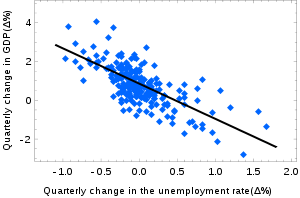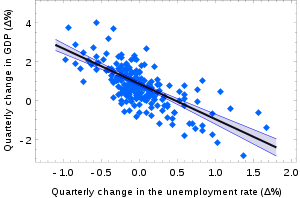# Simple linear regressionOkun's law in macroeconomics is an example of the simple linear regression. Here the dependent variable (GDP growth) is presumed to be in a linear relationship with the changes in the unemployment rate.

In statistics, simple linear regression is a linear regression model with a single explanatory variable. That is, it concerns two-dimensional sample points with one independent variable and one dependent variable (conventionally, the x and y coordinates in a Cartesian coordinate system) and finds a linear function (a non-vertical straight line) that, as accurately as possible, predicts the dependent variable values as a function of the independent variables. The adjective simple refers to the fact that the outcome variable is related to a single predictor.

It is common to make the additional hypothesis that the ordinary least squares method should be used to minimize the residuals. Under this hypothesis, the accuracy of a line through the sample points is measured by the sum of squared residuals (vertical distances between the points of the data set and the fitted line), and the goal is to make this sum as small as possible. Other regression methods that can be used in place of ordinary least squares include least absolute deviations (minimizing the sum of absolute values of residuals) and the Theil–Sen estimator (which chooses a line whose slope is the median of the slopes determined by pairs of sample points). Deming regression (total least squares) also finds a line that fits a set of two-dimensional sample points, but (unlike ordinary least squares, least absolute deviations, and median slope regression) it is not really an instance of simple linear regression, because it does not separate the coordinates into one dependent and one independent variable and could potentially return a vertical line as its fit.

The remainder of the article assumes an ordinary least squares regression. In this case, the slope of the fitted line is equal to the correlation between y and x corrected by the ratio of standard deviations of these variables. The intercept of the fitted line is such that it passes through the center of mass (x, y) of the data points.

## Fitting the regression line

Suppose there are n data points {(xi, yi), i = 1, ..., n}. The function that describes x and y is:The goal is to find the equation of the straight linewhich would provide a "best" fit for the data points. Here the "best" will be understood as in the least-squares approach: a line that minimizes the sum of squared residuals of the linear regression model. In other words, α (the y-intercept) and β (the slope) solve the following minimization problem:By simply expanding to get a quadratic expression in α and β, it can be shown that the values of α and β that minimize the objective function Q arewhere rxy is the sample correlation coefficient between x and y; and sx and sy are the sample standard deviation of x and y. A horizontal bar over a quantity indicates the average value of that quantity. For example:Substituting the above expressions forandintoyieldsThis shows that rxy is the slope of the regression line of the standardized data points (and that this line passes through the origin).

It is sometimes useful to calculate rxy from the data independently using this equation:The coefficient of determination (R squared) is equal towhen the model is linear with a single independent variable. See sample correlation coefficient for additional details.

### Linear regression without the intercept term

Sometimes it is appropriate to force the regression line to pass through the origin, because x and y are assumed to be proportional. For the model without the intercept term, y = βx, the OLS estimator for β simplifies toSubstituting (xh, yk) in place of (x, y) gives the regression through (h, k):The last form above demonstrates how moving the line away from the center of mass of the data points affects the slope.

## Numerical properties

1. The regression line goes through the center of mass point,, if the model includes an intercept term (i.e., not forced through the origin).
2. The sum of the residuals is zero if the model includes an intercept term:3. The residuals and x values are uncorrelated, meaning (whether or not there is an intercept term in the model):## Model-cased properties

Description of the statistical properties of estimators from the simple linear regression estimates requires the use of a statistical model. The following is based on assuming the validity of a model under which the estimates are optimal. It is also possible to evaluate the properties under other assumptions, such as inhomogeneity, but this is discussed elsewhere.

### Unbiasedness

The estimatorsandare unbiased. This requires that we interpret the estimators as random variables and so we have to assume that, for each value of x, the corresponding value of y is generated as a mean response α + βx plus an additional random variable ε called the error term. This error term has to be equal to zero on average, for each value of x. Under such interpretation, the least-squares estimatorsandwill themselves be random variables, and they will unbiasedly estimate the "true values" α and β.

### Confidence intervals

The formulas given in the previous section allow one to calculate the point estimates of α and β — that is, the coefficients of the regression line for the given set of data. However, those formulas don't tell us how precise the estimates are, i.e., how much the estimatorsandvary from sample to sample for the specified sample size. Confidence intervals were devised to give a plausible set of values the estimates might have if one repeated the experiment a very large number of times.

The standard method of constructing confidence intervals for linear regression coefficients relies on the normality assumption, which is justified if either:

1. the errors in the regression are normally distributed (the so-called classic regression assumption), or
2. the number of observations n is sufficiently large, in which case the estimator is approximately normally distributed.

The latter case is justified by the central limit theorem.

### Normality assumption

Under the first assumption above, that of the normality of the error terms, the estimator of the slope coefficient will itself be normally distributed with mean β and variancewhere σ2 is the variance of the error terms (see Proofs involving ordinary least squares). At the same time the sum of squared residuals Q is distributed proportionally to χ2 with n − 2 degrees of freedom, and independently from. This allows us to construct a t-statisticwhereis the standard error of the estimator.

This t-statistic has a Student's t-distribution with n − 2 degrees of freedom. Using it we can construct a confidence interval for β:at confidence level (1 − γ), whereis thequantile of the tn−2 distribution. For example, if γ = 0.05 then the confidence level is 95%.

Similarly, the confidence interval for the intercept coefficient α is given byat confidence level (1 − γ), whereThe US "changes in unemployment – GDP growth" regression with the 95% confidence bands.

The confidence intervals for α and β give us the general idea where these regression coefficients are most likely to be. For example, in the Okun's law regression shown at the beginning of the article the point estimates areThe 95% confidence intervals for these estimates areIn order to represent this information graphically, in the form of the confidence bands around the regression line, one has to proceed carefully and account for the joint distribution of the estimators. It can be shown that at confidence level (1 − γ) the confidence band has hyperbolic form given by the equation### Asymptotic assumption

The alternative second assumption states that when the number of points in the dataset is "large enough", the law of large numbers and the central limit theorem become applicable, and then the distribution of the estimators is approximately normal. Under this assumption all formulas derived in the previous section remain valid, with the only exception that the quantile t*n−2 of Student's t distribution is replaced with the quantile q* of the standard normal distribution. Occasionally the fraction 1/n−2 is replaced with 1/n. When n is large such a change does not alter the results appreciably.

## Numerical example

This example concerns the data set from the ordinary least squares article. This data set gives average masses for women as a function of their height in a sample of American women of age 30–39. Although the OLS article argues that it would be more appropriate to run a quadratic regression for this data, the simple linear regression model is applied here instead.

 Height (m), xi Mass (kg), yi 1.47 1.5 1.52 1.55 1.57 1.6 1.63 1.65 1.68 1.7 1.73 1.75 1.78 1.8 1.83 52.21 53.12 54.48 55.84 57.2 58.57 59.93 61.29 63.11 64.47 66.28 68.1 69.92 72.19 74.46

There are n = 15 points in this data set. Hand calculations would be started by finding the following five sums:These quantities would be used to calculate the estimates of the regression coefficients, and their standard errors.The 0.975 quantile of Student's t-distribution with 13 degrees of freedom is t*13 = 2.1604, and thus the 95% confidence intervals for α and β areThe product-moment correlation coefficient might also be calculated:This example also demonstrates that sophisticated calculations will not overcome the use of badly prepared data. The heights were originally given in inches, and have been converted to the nearest centimetre. Since the conversion factor is one inch to 2.54 cm, this is not a correct conversion. The original inches can be recovered by Round(x/0.0254) and then re-converted to metric: if this is done, the results becomeThus a seemingly small variation in the data has a real effect.

## Derivation of simple regression estimators

We look forandthat minimize the sum of squared errors (SSE):To find a minimum take partial derivatives with respect toandBefore taking partial derivative with respect to, substitute the previous result for.Now, take the derivative with respect to:And finally substituteto determine